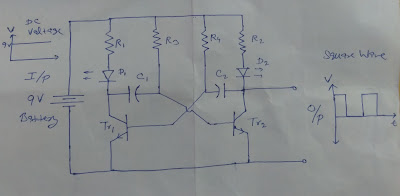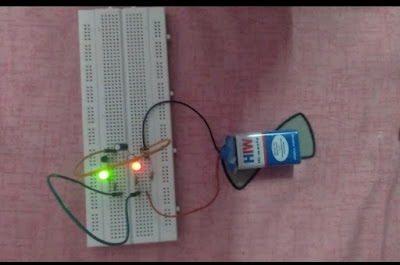An astable multi-vibrator is an electronic circuit (consists of resistors, capacitors, and transistors) that produce sustained oscillations of fixed frequency depending upon the time constant of the circuit. It is also called a square wave generator circuit.

#### Components Required:-

To make an astable multi-vibrator circuit following Components are required...

1. Resistors:- two 1kΩ 1/4 watt and two 10kΩ 1/4 watt
2. Transistors:- two npn transistor BC-547
3. Capacitors:- two 100 μF, 25 volt
4. LED :- two colored LED s
5. Battery:- 9 V battery
7. Jumper wires

#### Circuit Diagram:-

In Fig.1 Astable multivibrator circuit diagram' 1kΩ, 1/4 watt resistors are used to provide limited voltage and current to LED s. A LED normally takes 1.5 to 2.5 V forward voltage and 5 to 20 mA forward current to produce light.  Intensity of light depends upon amount of forward current. One can find out value of forward  current through LED as below

If = [ Battery Voltage (9 V) - LED Voltage drop (1.5 V ) ] ÷ Resistance Value (1kΩ )

If  = 7.5 mA

Please note that intensity of LED light  can be increased by decreasing resistance value. To increase forward current upto 20 mA resistance value  must be decreased upto 375 Ω but as this value resistance is not available so 470 Ω resistance can be used or can be made using a parellel or series combination of resistors.

10 kΩ 1/4 watt resistors are used to discharge the capacitors charge. npn transistors are used in saturation and cut off mode to act as switches. Capacitors control the switching frequency of transistors and help in producing square wave at the output of astable multivibrator circuit, which can be seen by LEDs blinking and Cathode Ray Oscilloscope.Fig.1 Astable multivibrator circuit diagram

#### Circuit Operation :-

When 9 V supply is given to circuit, current flow through both 1kΩ, 1/4 watt resistors to LEDs and as in starting capacitors act as short circuit current goes to transistors base. The transistor which operates in saturation region first that branch LED starts to glow where as other LED remains off. But as capacitor get fully charged it becomes open circuited and at that time another transistor goes into saturation region and that branch LED starts to glow. Charged capacitors get discharged through 10 kΩ 1/4 watt resistors and the process goes on. As long as this process goes on both LED s keep blinking. Depending upon the time constant of the circuit LED starts blinking.Fig. 2 When Green LED is glowing